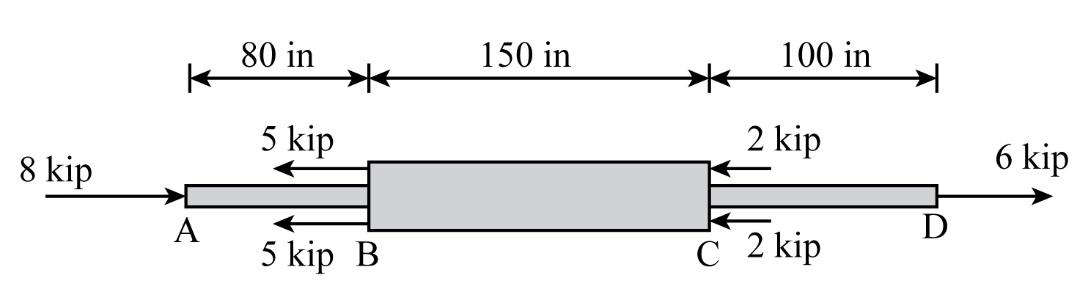×
Log in to StudySoup
Get Full Access to Mechanics Of Materials - 10 Edition - Chapter 4 - Problem 4-2
Join StudySoup for FREE
Get Full Access to Mechanics Of Materials - 10 Edition - Chapter 4 - Problem 4-2

Already have an account? Login here
×
Reset your password

# Solved: The copper shaft is subjected to the axial loadsISBN: 9780134319650 134

## Solution for problem 4-2 Chapter 4

Mechanics of Materials | 10th Edition

• Textbook Solutions
• 2901 Step-by-step solutions solved by professors and subject experts
• Get 24/7 help from StudySoup virtual teaching assistantsMechanics of Materials | 10th Edition

4 5 1 269 Reviews
27
5
Problem 4-2

The copper shaft is subjected to the axial loads shown. Determine the displacement of end A with respect to end D if the diameters of each segment are dAB = 0.75 in., dBC = 1 in., and dCD = 0.5 in. Take Ecu = 18(103) ksi.

Step-by-Step Solution:

Problem 4-2

The copper shaft is subjected to the axial loads shown. Determine the displacement of end A with respect to end D if the diameters of each segment are,and. Take.Step by step solution

Step 1 of 3

The diameter is.

The diameter is.

The diameter is.

In order to determine the total displacement, we need to determine the displacement in segment AB.Substitute the given values,,andin the above expression.Step 2 of 3

Step 3 of 3

##### ISBN: 9780134319650

This full solution covers the following key subjects: end, axial, dab, dbc, dcd. This expansive textbook survival guide covers 14 chapters, and 1373 solutions. The answer to “The copper shaft is subjected to the axial loads shown. Determine the displacement of end A with respect to end D if the diameters of each segment are dAB = 0.75 in., dBC = 1 in., and dCD = 0.5 in. Take Ecu = 18(103) ksi.” is broken down into a number of easy to follow steps, and 46 words. Since the solution to 4-2 from 4 chapter was answered, more than 373 students have viewed the full step-by-step answer. This textbook survival guide was created for the textbook: Mechanics of Materials, edition: 10. Mechanics of Materials was written by and is associated to the ISBN: 9780134319650. The full step-by-step solution to problem: 4-2 from chapter: 4 was answered by , our top Engineering and Tech solution expert on 11/10/17, 06:06PM.

Unlock Textbook Solution

Enter your email below to unlock your verified solution to:

Solved: The copper shaft is subjected to the axial loads